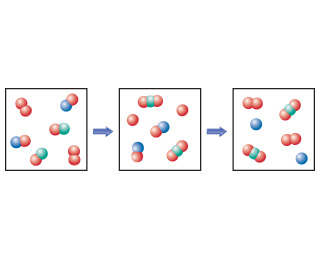# Problem: The following diagram represents an imaginary two-step mechanism. Let the red spheres represent element A, the green ones element B, and the blue ones element C.Identify the catalyst.

###### FREE Expert Solution

In this problem, we are asked to identify the catalyst in the given figure.

Recall that catalyst is any substance that increases the rate of a reaction without itself being consumed.###### Problem Details

The following diagram represents an imaginary two-step mechanism. Let the red spheres represent element A, the green ones element B, and the blue ones element C.Identify the catalyst.